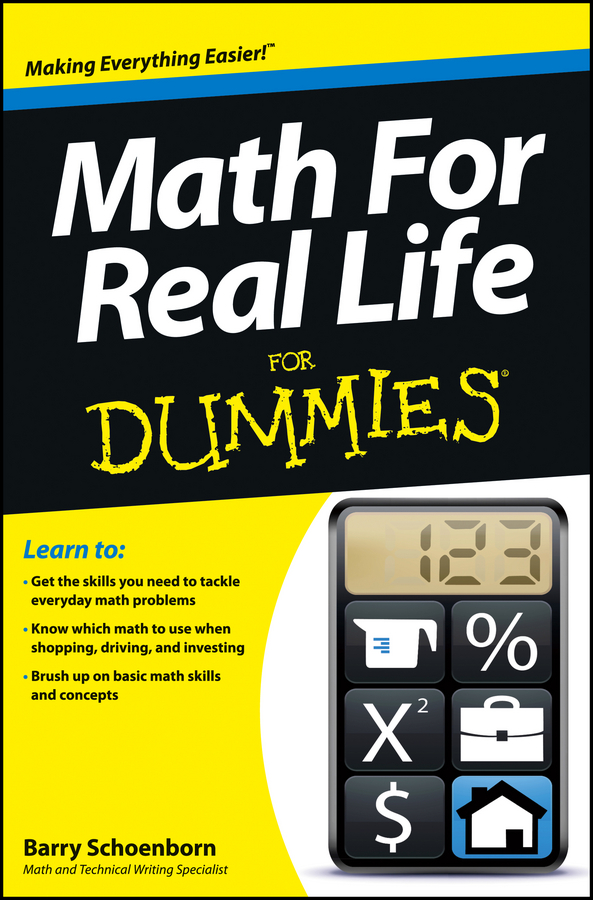##### Math For Real Life For DummiesPart of making maths easier to understand is explaining what the different terms mean. Here’s a jargon-busting guide to help you along with maths questions involving shapes, space and measures.

• Acute angle: An angle smaller than a right-angle – so less than 90 degrees.

• Angle: The sharpness of a corner, measured in degrees. If you turn all the way around to face the way you started, you move through 360 degrees.

• Area: How much of something, such as paper, you need to cover a surface. You use different formulas to work out area for different shapes. You measure area in centimetres squared (cm2), or metres squared (m2) or any other unit of length squared.

• Capacity: How much space there is inside a shape.

• Circle: This is the only curved shape you really need to know about. The technical definition is ‘a shape with all of the points a fixed distance from the centre’, but you’ll recognise a circle when you see one.

• Cube: The shape of a normal die. Each of a cube’s sides is a square, and all of the edges are the same length.

• Cuboid: The shape of a shoebox. Each of its sides is a rectangle.

• Distance: How far one thing is from another. You measure distance in units of length, such as centimetres, metres, feet or inches.

• Length: How long an object is. You normally measure length in centimetres, metres, feet or inches.

• Obtuse angle: An angle that’s more than 90 degrees but less than 180.

• Perimeter: How far around a shape is – that is, if you walked all the way around a shape, how far you would travel. You measure perimeter in units of length, such as centimetres, metres, feet or inches. You find the answer by adding up the length of all of a shape’s sides.

• Pyramid: The shape of . . . well, guess! It has a square on the bottom and four identical triangles around the side that come together and meet at the top.

• Rectangle: A shape with four straight sides at right-angles to each other. The sides aren’t necessarily all the same length, but sides opposite each other are always the same length.

• Reflection: Turning a shape over.

• Reflex angle: An angle bigger than 180 degrees.

• Regular: A regular shape is one where all the sides are the same length and all the corners have the same angle.

• Right-angle: A quarter-turn, or 90 degrees.

• Rotation: Twisting a shape around its centre.

• Sphere: The shape of a ball.

• Square: A shape with four equal-length straight sides arranged at right-angles to each other. Again, you’ll probably recognise a square when you see one!

• Symmetry: A symmetrical shape is one you can either fold precisely in half, or twist around its centre so you can’t tell which way up it is.

• Temperature: How hot (or cold) something is, normally measured in degrees Celsius.

• Tessellation: Putting identical shapes together to make a pattern, with no spaces between the shapes.

• Triangle: Yes, you guessed it, a shape with three straight sides.

• Volume: How much space a shape takes up in three dimensions (3D).

• Weight: How heavy something is, normally measured in kilograms or grams.